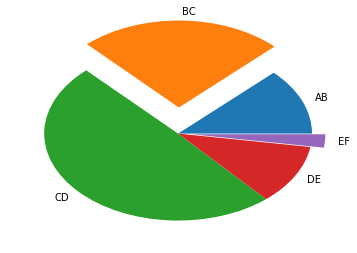### Plot A Basic PieChart Using MATPLOTLIB In Python

Objective: To make a basic pie chart in python using its one of the most famous library for visualization Matplotlib.pyplot.

Download the code file

#Import Libraries
>import numpy as np
>import matplotlib.pyplot as mat

# Make a basic Pie chart
>data = [10,20,40,9,2]
>mat.pie(data)
>mat.show()

### Output:# Add labels to your Pie Chart

>data = [10,20,40,9,2]
>labels = ['AB', 'BC', 'CD', 'DE', 'EF']
>mat.pie(data, labels = labels)
>mat.show()

### Output:# Explode a pie
>data = [10,20,40,9,2]
>explode = [0,0.3,0,0,0.1]
>labels = ['AB', 'BC', 'CD', 'DE', 'EF']
>mat.pie(data, labels = labels, explode = explode)
>mat.show()

### Output:# Add a shadow or change the direction of the pie chart

>data = [10,20,40,9,2]
>explode = [0,0.3,0,0,0.1]
>labels = ['AB', 'BC', 'CD', 'DE', 'EF']
>mat.pie(data, explode = explode, labels = labels, shadow = True,counterclock = False)
>mat.show()